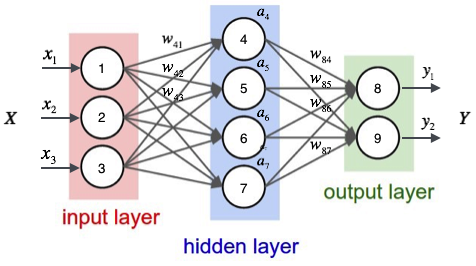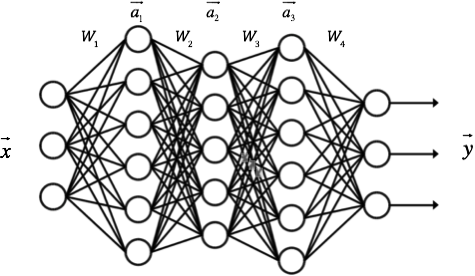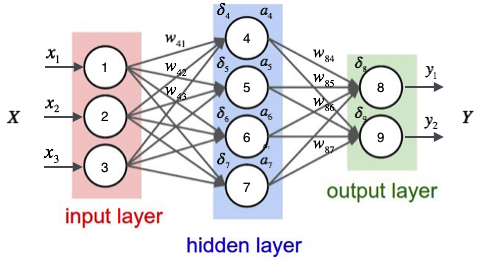# 0x00 神经元

$\text{sigmoid}(x)=\frac{1}{1+e^{-x}}$

$y'=y(1-y)$$w_{41}$

$a_4$

$a_4=\text{sigmoid}(w_{41}x_1+w_{42}x_2+w_{43}x_3+b_4)$

$w_{4b}$

$a_4=\text{sigmoid}(w_{41}x_1+w_{42}x_2+w_{43}x_3+w_{4b})$

$a_4=\text{sigmoid}([w_{41},w_{42},w_{43},w_{4b}]\begin{bmatrix}x_1\\x_2\\x_3\\1\end{bmatrix}) \\ 令\vec{w_4}=[w_{41},w_{42},w_{43},w_{4b}], \vec{x}=\begin{bmatrix}x_1\\x_2\\x_3\\1\end{bmatrix}\\ 则有a_4=\text{sigmoid}(\vec{w_4}\cdot \vec{x})$

$\vec{a}=\begin{bmatrix} a_4 \\ a_5 \\ a_6 \\a_7 \end{bmatrix}=\text{sigmoid}\left( \begin{bmatrix} w_{41}&w_{42}&w_{43}&w_{4b} \\ w_{51}&w_{52}&w_{53}&w_{5b} \\ w_{61}&w_{62}&w_{63}&w_{6b} \\ w_{71}&w_{72}&w_{73}&w_{7b} \end{bmatrix} \begin{bmatrix}x_1\\x_2\\x_3\\1\end{bmatrix} \right)$

$W_1$
，由此，我们就可以得到：
$\vec{a}=\text{sigmoid}(W_1\cdot \vec{x})$

\begin{align} \vec{y}=\begin{bmatrix} y_1 \\ y_2 \end{bmatrix} =& \text{sigmoid}\left( \begin{bmatrix} w_{84}a_4+w_{85}a_5+w_{86}a_6+w_{87}a_7+w_{8b} \\ w_{94}a_4+w_{95}a_5+w_{96}a_6+w_{97}a_7+w_{9b} \end{bmatrix} \right) \\ =& \text{sigmoid}\left( \begin{bmatrix} w_{84}&w_{85}&w_{86}&w_{87}&w_{8b} \\ w_{94}&w_{95}&w_{96}&w_{97}&w_{9b} \end{bmatrix} \begin{bmatrix} a_4 \\ a_5 \\ a_6 \\a_7 \\ 1 \end{bmatrix} \right) \\ =& \text{sigmoid}\left( W_2\cdot \vec{a} \right) \\ \end{align}\begin{align} \vec{a}_1&=f(W_1\cdot\vec{x})\\ \vec{a}_2&=f(W_2\cdot\vec{a_1})\\ \vec{a}_3&=f(W_3\cdot\vec{a_2})\\ \vec{y}&=f(W_4\cdot\vec{a_3})\\ \end{align}

# 0x01 反向传播算法

$E_d\equiv\frac{1}{2}\sum_{i\in \text{outputs}}(t_i-y_i)^2$

$w_{ji}\gets w_{ji}-\eta\frac{\partial{E_d}}{\partial{w_{ji}}}$

$E_d$

$w_{ji}$$w_{ji}$

$i$

$j$

$w_{ji}$

$j$

$w_{41}$

$\text{net}_j$

$j$

$\text{net}_j=\sum_i w_{ji}\cdot x_{ji}$

\begin{align} \frac{\partial{E_d}}{\partial{w_{ji}}}&=\frac{\partial{E_d}}{\partial{\text{net}_j}}\frac{\partial{\text{net}_j}}{\partial{w_{ji}}}\\ &=\frac{\partial{E_d}}{\partial{\text{net}_j}}\frac{\partial}{\partial{w_{ji}}}\left(\sum_{i}{w_{ji}}x_{ji}\right)\\ &=\frac{\partial{E_d}}{\partial{\text{net}_j}}x_{ji} \end{align}

$w_{ji}$

$w_{j(i-1)}$
，对
$w_{ji}$

$x_{ji}$

$\frac{\partial{E_d}}{\partial{\text{net}_j}}$

$E_d$

$\text{net}_j$

$y_j$

$E_d\equiv\frac{1}{2}\sum_{i\in \text{outputs}}(t_i-y_i)^2$
$E_d$
$y_i$

$y_i$
$\text{net}_j$

\begin{align} \frac{\partial{E_d}}{\partial{\text{net}_j}}&=\frac{\partial{E_d}}{\partial{y_j}}\frac{\partial{y_j}}{\partial{\text{net}_j}}\\ \end{align}

\begin{align} \frac{\partial{E_d}}{\partial{y_j}}&=\frac{\partial}{\partial{y_j}}\left(\frac{1}{2}\sum_{i\in \text{outputs}}(t_i-y_i)^2\right)\\ &=\frac{\partial}{\partial{y_j}}\left(\frac{1}{2}(t_j-y_j)^2\right)\\ &=-(t_j-y_j) \end{align}

\begin{align} \frac{\partial{y_j}}{\partial{\text{net}_j}}&=\frac{\partial \text{sigmoid}(\text{net}_j)}{\partial{\text{net}_j}}=y_j(1-y_j) \end{align}

$\frac{\partial{E_d}}{\partial{\text{net}_j}}=-y_j(t_j-y_j)(1-y_j)$

$\frac{\partial{Ed}}{\partial{w{ji}}}$
，解得：
\begin{align} \frac{\partial{E_d}}{\partial{w_{ji}}}&=\frac{\partial{E_d}}{\partial{\text{net}_j}}x_{ji}=-y_j(t_j-y_j)(1-y_j)x_{ji} \end{align}
$\delta_j=-\frac{\partial{E_d}}{\partial{\text{net}_j}}$
，也就是一个结点的误差项
$\delta$

$a = b$

$\text{net}_j$

$\delta_j=y_j(t_j-y_j)(1-y_j)$

\begin{align} w_{ji}&\gets w_{ji}-\eta\frac{\partial{E_d}}{\partial{w_{ji}}}\\ &=w_{ji}+\eta(t_j-y_j)y_j(1-y_j)x_{ji}\\ &=w_{ji}+\eta\delta_jx_{ji} \end{align}

$j$

$j$

$E_d$
。由此我们定义节点
$j$

$\text{Downstream}(j)$
，设
$\text{net}_k$

$j$

$j$

$\text{net}_k$
$\text{net}_j$

$E_d$

$\text{net}_k$

\begin{align} \frac{\partial{E_d}}{\partial{\text{net}_j}}&=\sum_{k\in \text{Downstream}(j)}\frac{\partial{E_d}}{\partial{\text{net}_k}}\frac{\partial{\text{net}_k}}{\partial{\text{net}_j}}\\ &=\sum_{k\in \text{Downstream}(j)}-\delta_k\frac{\partial{\text{net}_k}}{\partial{\text{net}_j}}\\ &=\sum_{k\in \text{Downstream}(j)}-\delta_k\frac{\partial{\text{net}_k}}{\partial{a_j}}\frac{\partial{a_j}}{\partial{\text{net}_j}}\\ &=\sum_{k\in \text{Downstream}(j)}-\delta_kw_{kj}\frac{\partial{a_j}}{\partial{\text{net}_j}}\\ &=\sum_{k\in \text{Downstream}(j)}-\delta_kw_{kj}a_j(1-a_j)\\ &=-a_j(1-a_j)\sum_{k\in \text{Downstream}(j)}\delta_kw_{kj} \end{align}

$a_j$

$j$

$a_j= \text{sigmoid}(Wx)$

$a_j=\text{sigmoid}(\text{net}_j)$

$\text{net}_{k}$

$\text{net}_{k}=w_{kj}\cdot a_j$

$\delta_j=-\frac{\partial{E_d}}{\partial{\text{net}_j}}$

$\delta_j=a_j(1-a_j)\sum_{k\in \text{Downstream}(j)}\delta_kw_{kj}$$\delta_8$

\begin{align} \delta_8=& y_1(t_1-y_1)(1-y_1) \end{align}

$\delta_4$

$\delta_4=a_4(1-a_4)(\delta_8w_{84}+\delta_9w_{94})$

$w_{ji}\gets w_{ji}+\eta\delta_jx_{ji}$

# 0x02 检查神经网络是否出错

$w_{ji}\gets w_{ji}-\eta\frac{\partial{E_d}}{\partial{w_{ji}}}$

$\frac{\partial{E_d}}{\partial{w_{ji}}}$

$f'(x)=\lim_{\epsilon \to 0}\frac{f(x+\epsilon)-f(x-\epsilon)}{2\epsilon}$

$\epsilon$

\begin{align} \frac{\partial{E_d}}{\partial{w_{ji}}}&=\lim_{\epsilon \to 0}\frac{f(w_{ji}+\epsilon)-f(w_{ji}-\epsilon)}{2\epsilon} \\ &\approx \frac{f(w_{ji}+\epsilon)-f(w_{ji}-\epsilon)}{2\epsilon} \end{align}

# 0x04 为什么没有TensorFlow跑得快

• 全部使用python编写，语言本身效率低，而TensorFlow底层计算全部基于C++编写的动态链接库，TensorFlow python端仅是一个用于与底层库交互的接口，实际的计算工作全部都是在底层库中完成的。
• 全部基于CPU计算，TensorFlow GPU版可以完成基于GPU的计算，众所周知，GPU的浮点计算速度远高于CPU。
• 没有使用向量化编程，没必要定义太多复杂的对象，直接把数学计算实现了就可以了。
• 没有使用SGD（随机梯度下降算法）